Geometry

# Regular Polygons Warmup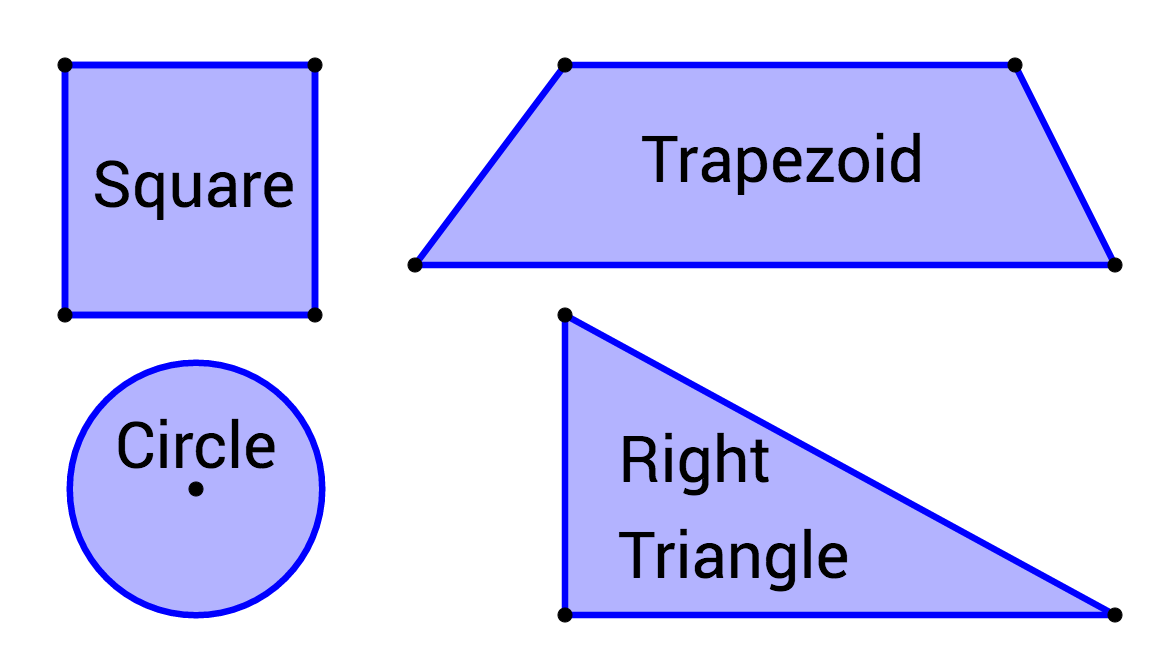Which shape is a regular polygon?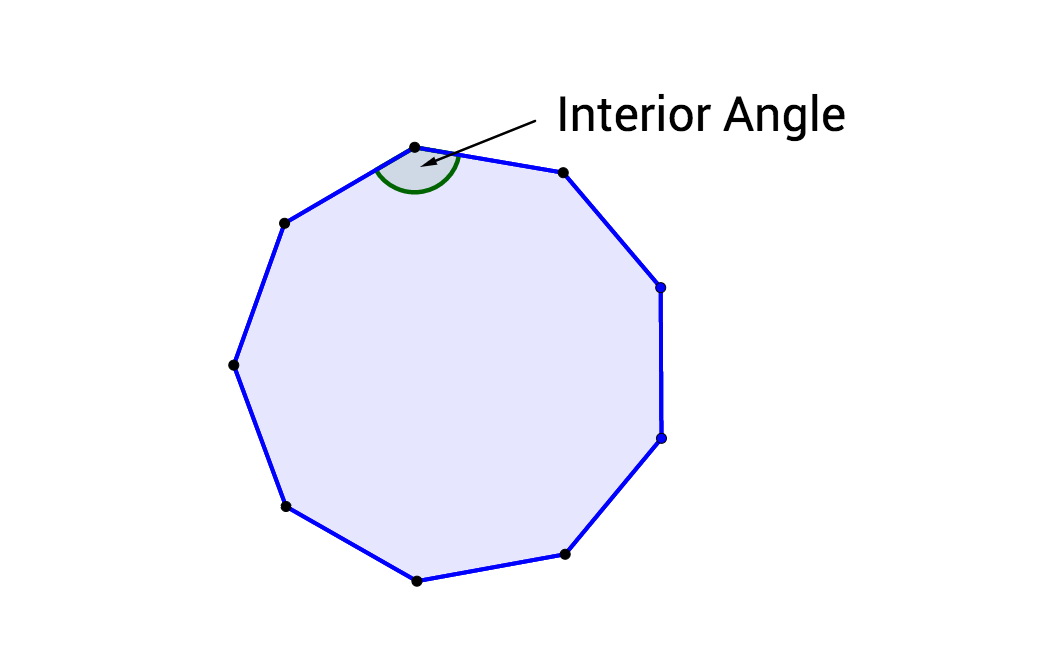If the sum of the interior angles in a polygon equals $$1080^\circ,$$ how many sides does the polygon have? (Hint: A triangle has an interior angle sum of $$180^\circ.$$ A quadrilateral has an interior angle sum of $$360^\circ.$$ A pentagon has an interior angle sum of $$540^\circ.$$)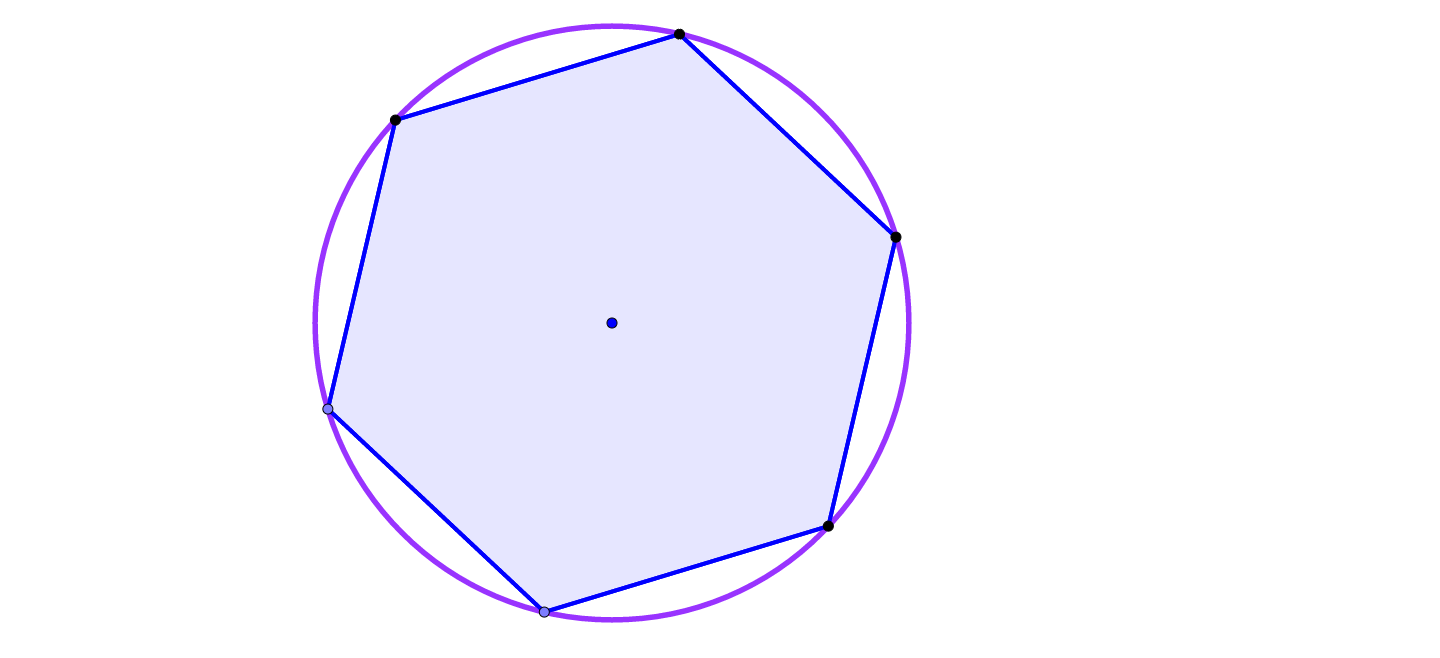A hexagon is inscribed in a circle with a radius of 6. What is the area of the hexagon?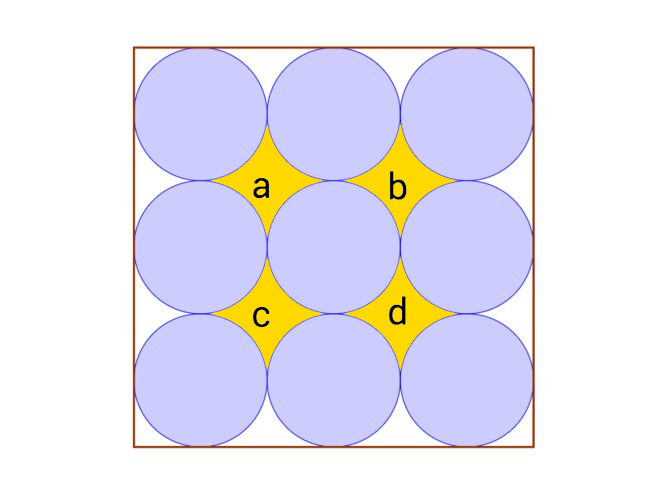Nine identical circles are placed adjacent to one another in three rows in a square whose area is 9. $$a, b, c,$$ and $$d$$ represent the areas of those regions between the circles. What is $$a + b + c + d?$$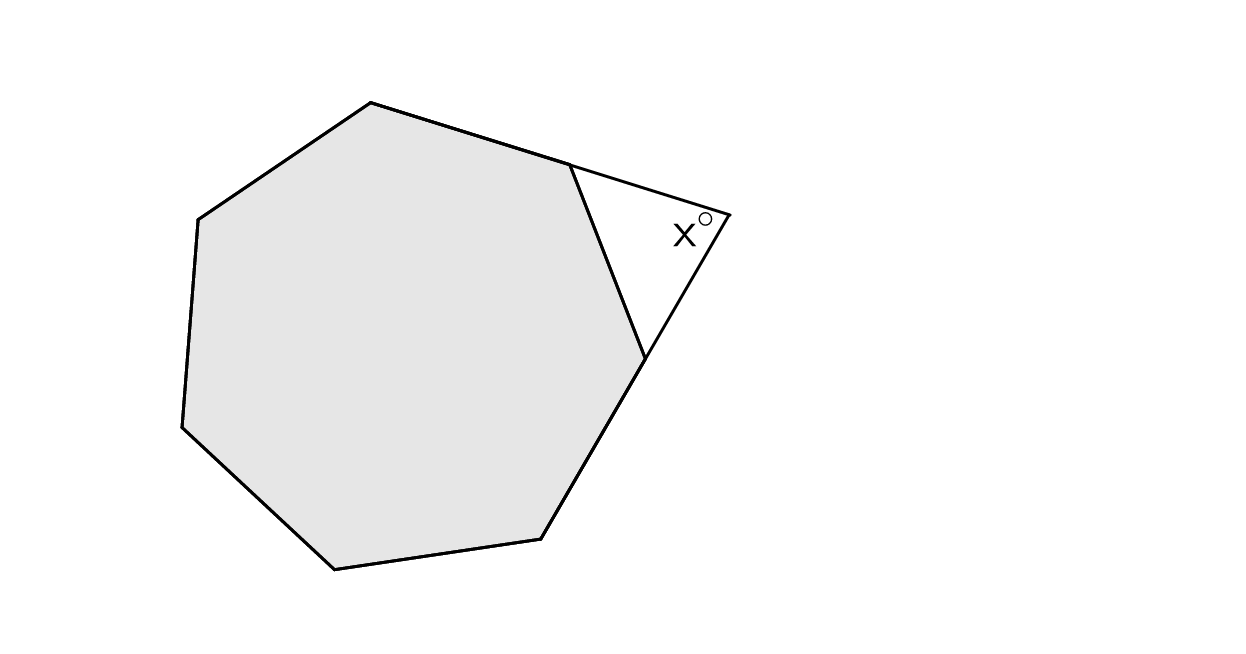An angle is formed by extending two sides of a regular heptagon, as shown. What is the closest approximate value of $$x?$$

×